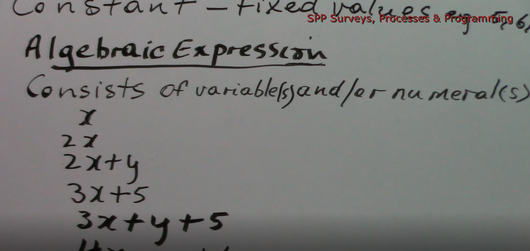Algebra - Introduction (Part 1)

Regular price \$2.00 Sale

Introduction to Algebra Part 1 Tutorial done by M. Chan (Trained Certified Teacher).

Topics covered in this 30:11 minute video tutorial (1.53GB video file) are:

1 ) Variables

2) Constants

3) Terms / Expression (Coefficient, Base and Exponents)

4) Multiplication

5) Division

6) Changing Written Expression to Algebric Expression

7) Substitution